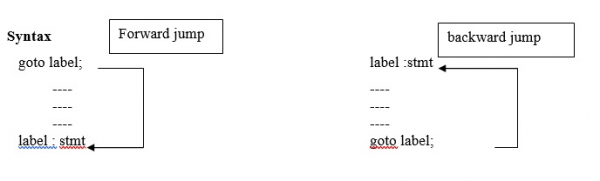# C program to explain the goto statement

CServer Side ProgrammingProgramming

The C program evaluates the square root for five numbers. The variable count stores the count of numbers read. When count is less than or equal to 5, goto read statement directs the control to the label read. Otherwise, the program prints a message and stops.

## Goto statement

It is used after the normal sequence of program execution by transferring the control to some other part of program.## Program

Following is the C program for usage of goto statement −

#include <math.h>
main(){
double x, y;
int count;
count = 1;
printf("Enter FIVE real values in a LINE \n");
scanf("%lf", &x);
printf("\n");
if (x < 0)
printf("Value - %d is negative\n",count);
else{
y = sqrt(x);
printf("%lf\t %lf\n", x, y);
}
count = count + 1;
if (count <= 5)
printf("\nEnd of computation");
}

## Output

When the above program is executed, it produces the following result −

Enter FIVE real values in a LINE
2.3 -4.5 2 6.8 -44.7
2.300000 1.516575
Value - 2 is negative
2.000000 1.414214
6.800000 2.607681
Value - 5 is negative
End of computation